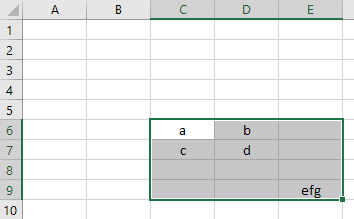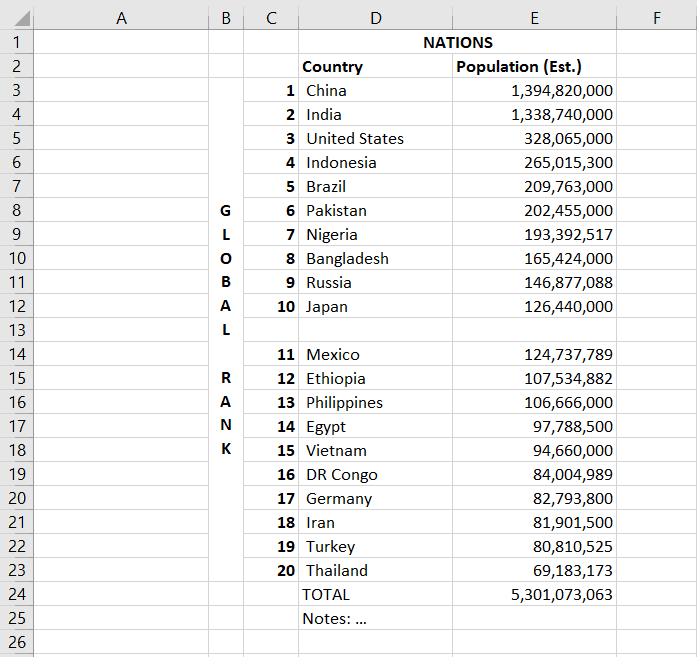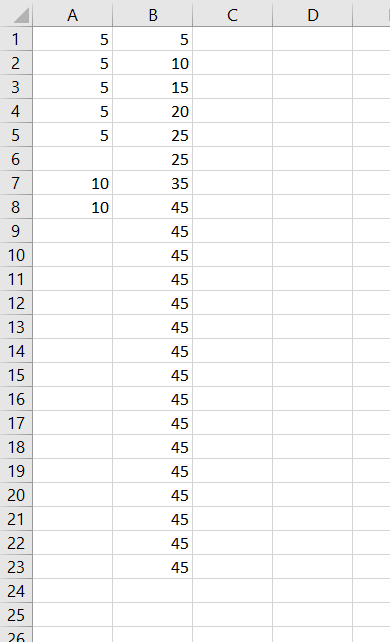The VBA UsedRange property is a neat property to add to your VBA repertoire. It’s commonly found in internet threads for finding the count of rows and columns on a sheet - and by proxy the iteration counts for for-loops - and it’s certainly useful for that. `UsedRange` has some other interesting properties, too, and there are a handful of warnings to keep in mind. Let’s check it out.

## `UsedRange` Object

`UsedRange` is a special Range property that specifies the range of cells on a worksheet that have been, well, used. Truth is, certain modifications to a cell also designate it as “used” according to VBA. We’ll talk about this more in a few minutes, but a better description of the VBA UsedRange property is a range of cells, on the specified sheet, that have content, formatting, comments, or certain other modifications. It’s a great way to gain better control of your spreadsheet’s data.

Since `UsedRange` is a property of the Range object, all of the properties and methods available to Ranges are also available to `UsedRange`. This includes column/row counts, selecting, clearing, formatting, and range navigation. Moreover, you can set it as a named object for more convenient reference, like so:

``````Dim rng As Range
Set rng = Sheets("targetSheet").UsedRange``````

where targetSheet is the name of the sheet you want to work with.

Setting a named object, like rng, lets you access Intellisense, an invaluable tool. Be aware that objects are not updated on-the-fly, so you need to set it again to get updated properties! If you set rng then change anything, like applying the `.Clear` method, your variable rng will NOT update automatically. You’ll need to set it again.

To demonstrate what VBA UsedRange actually does, add a couple values to an empty spreadsheet and run this short macro:

``````Sub SelectUsedRange()
ActiveSheet.UsedRange.Select
End Sub``````

This macro selects the used range on your spreadsheet. You can see that VBA attempts to calculate the first cell and the last cell with data on your spreadsheet and selects everything in that range. Notice in the image below that any empty cells in the used range are also included when using the UsedRange property. Even though you might think it would, the VBA UsedRange property doesn’t just capture the union of all cells containing data.## The Shape of the Range

Like we just demonstrated, VBA does not give you a choice in the shape of the range covered by `UsedRange`. It always produces a rectangle and starts in the upper leftmost corner and fans out to capture the lowest row and the rightmost column. Even though they define the boundaries of UsedRange, the upper left and lower right cells may not actually contain any values.

For example, the `UsedRange` in this image is B1:E25, even though the four cells in the top corner, including cell B1, contain no data or formatting. Neither does cell E25. The same is true for the four bottom left cells. Importantly, the blank row between the top ten and next ten entries, row 13, is also counted - keep this in mind when using VBA UsedRange as the basis for your macro’s row and column counting!UsedRange with Unfilled Cells

Make powerful spreadsheets with our free VBA Developer's Guide

Sure, I'll take a free VBA guide.

## Working with Rows and Columns

`UsedRange` is handy for finding the first and last rows and columns used on a worksheet. By extension, it can be used to count rows and columns to help iterate through for-loops.

### Using the `.Address` Property

One way to get the first row/column pair and last row/column pair is to use the `.Address` property of the UsedRange:

``````Dim rng As Range
Set rng = Sheets("targetSheet").UsedRange

This returns the string \$B\$1:\$E\$25, which can be parsed into the necessary pieces for the first row (1), the first column (B), the last row (25), and the last column (E). Recall that Debug.Print prints to your VBA Immediate Window.

### First Row and First Column

You can also easily find the first row and first column as a number using the `.Row` and `.Column` properties:

``````Dim rng As Range
Set rng = Sheets("targetSheet").UsedRange
Debug.Print rng.Row
Debug.Print rng.Column``````

This simply returns the first row number and first column number of the range, (1 and 2 in our example), which corresponds to the \$B\$1 cell that we talked about earlier.

The number for the last row and column requires a bit more work. One way to get the last row and column of a UsedRange is by using the `count` property.

## Counting Rows and Columns

To get the count of rows and columns in the range, you can use `.Count`

``````Dim rng As Range
Set rng = Sheets("targetSheet").UsedRange
Debug.Print rng.Rows.Count
Debug.Print rng.Columns.Count``````

This returns 25 and 4 in our example above.

### Last Row and Last Column

If the first cell in your UsedRange is \$A\$1, finding the last used row and column is simple since the `.Count` of the rows and columns equals your last used rows and columns.

``````Dim rng As Range
Set rng = Sheets("targetSheet").UsedRange
lastRow = rng.Rows.Count '25
lastCol = rng.Columns.Count '4``````

This often isn’t the case, though. If your data does not start at `\$A\$1`, this method will not work!

As can be seen in our example image, the last row is indeed Row 25. The last column, however, is not Column 4 (D). It’s Column 5 (E). To be more robust, you’d need to to add the first row/column numbers to the row/column counts.

``````Dim rng As Range
Set rng = Sheets("targetSheet").UsedRange
firstRow = rng.Row
firstCol = rng.Column

numRows = rng.Rows.Count
numCols = rng.Columns.Count

'remember to subtract 1 to not double-count the first row/column
lastRow = firstRow + numRows - 1
lastCol = firstCol + numCols - 1``````

Now we get our expected {25, 5} instead of {25, 4} result. Remember to subtract 1 from the final result, otherwise you’ll double-count the first row and column.

Now that we’ve demonstrated the logic for calculating the last used row and column in a UsedRange, let’s show you a much easier way. To record the last used row and column in a Used Range, simply use the following shortcut:

``````Dim rng As Range
Set rng = Sheets("targetSheet").UsedRange
lastRow = rng.Rows(rng.Rows.Count).Row
lastCol = rng.Columns(rng.Columns.Count).Column``````

### Counts for For-Loops

Notice our continued use of the `.Count` property. The `.Count` property is also useful when constructing your for-loops. Use the first row/column to start, then process an entire range down to the last row/column. For a simplified approach that only loops through the rows, let’s say a sheet only contained numeric data in Column A. You could store the running total of the numbers in Column B (Column 2) using a macro like this:

``````Sub RunningTotal()
Dim FirstRow As Integer, LastRow As Integer, iRow As Integer
Dim rng As Range
Set rng = ActiveSheet.UsedRange
FirstRow = rng.Row
LastRow = rng.Rows(rng.Rows.Count).Row

For iRow = FirstRow To LastRow
If iRow = FirstRow Then
Cells(iRow, 2) = Cells(iRow, 1) 'start off the running total
Else
Cells(iRow, 2) = Cells(iRow, 1) + Cells(iRow - 1, 2) 'add previous running total to new entry
End If
Next iRow
End Sub``````

You would need two nested loops to loop through both the rows and the columns in your used range, like this:

``````Sub LoopThroughUsedRange()
Dim FirstRow As Integer, LastRow As Integer
Dim FirstCol As Integer, LastCol As Integer
Dim iRow As Integer, iCol As Integer
Dim rng As Range
Set rng = ActiveSheet.UsedRange 'store the used range to a variable
FirstRow = rng.Row
FirstCol = rng.Column
LastRow = rng.Rows(rng.Rows.Count).Row
LastCol = rng.Columns(rng.Columns.Count).Column

For iCol = FirstCol To LastCol
For iRow = FirstRow To LastRow
Debug.Print Cells(iRow, iCol).Address & " = " & Cells(iRow, iCol)
Next iRow
Next iCol
End Sub``````

If you only want to process data to cells in the UsedRange that are not empty, apply the VBA IsEmpty function.

## Properties and Methods

Once you know how to navigate a UsedRange, applying properties using VBA is a breeze. Properties let you do things like change the entire range to bold font. Just note that the properties will be applied to the entire range, even if cells are blank. It would be tedious to go back and fix formatting for an entire sheet because of a single poorly thought out line of code.

``````Dim rng As Range
Set rng = Sheets("targetSheet").UsedRange
rng.Font.Bold = True``````

This macro snippet changes all cells on the worksheet containing data (or formatting plus empty cells between used cells) to bold. You can do the same thing with colors, cell fill colors, number formats, etc. Again, be aware the property will be applied to the entire used range, so if you have a nicely formatted table with 5000 cells, make sure you want all 5000 cells to be bold before running those lines of code!

### Clearing the Range

Another very common use of `UsedRange` is to clear the contents of a sheet. When using UsedRange, you don’t need to find the last row/column like we did above. After setting rng as a named object, simply execute:

``````Dim rng As Range
Set rng = Sheets("targetSheet").UsedRange
rng.Clear 'or rng.ClearContents to just clear data``````

Again, make sure you want to clear the entire worksheet of formats and data. It’s a little like using `sudo rm -r` in Linux: powerful and convenient, but risky.

Make powerful spreadsheets with our free VBA Developer's Guide

Sure, I'll take a free VBA guide.

## Navigating the Range

Ranges allow access relative to the range itself as opposed to relative to the worksheet. For example, in our table above, the first column is actually B, not A. If we knew the column layout of the table - axis name, rank, country, population - we could reference population relative to the sheet or relative to the range.

• population column relative to the sheet = 5
• population column relative to the used range = 4

Let’s imagine a user shifts the table to a different position on your worksheet for some reason. If you hardcoded your macro to grab population relative to the sheet, you may now be inadvertently grabbing the wrong data. It would be more robust to use the table’s own structure as the point of reference:

``````Dim rng As Range
Set rng = Sheets("targetSheet").UsedRange
rng.Columns(4).Font.Bold = True``````

If the user moves the table’s starting point to \$H\$15, for example, we can still bold the population column because the population column will still be the 4th column in our UsedRange.

## Hidden Changes

There’s at least one caveat to using `UsedRange` and it’s an important one to consider. The caveat deals with unseen changes. Cells that have been changed invisibly, such as with number formatting, font changes, or even comments, will be included in the coverage of UsedRange. What that means is if a user accidentally italicizes a cell without any data, that cell still counts for the “used range” so your UsedRange might be expanded beyond what you thought it would be. This expanded range would show up when selecting `UsedRange` and it would be included in the `.Count` and `.Address` properties.

This isn’t always a problem, but it can present easily-overlooked issues. In our RunningTotal example, if only the first 10 cells have data, one would expect to get the total up to Row 10. If, however, the user accidentally italicizes cell \$D\$23 with a slip on the keyboard and Ctrl+i, we’ll get this strange output in column B, which is bound to confuse the user:Format Changes Impacted VBA UsedRange

There is no obvious change to cell \$D\$23, but `UsedRange` considers the formatting change and includes the entire area from \$A\$1 to \$D\$23.

`UsedRange` makes it easy to find the first row/column and to apply formatting to any useful cells on a sheet. It also makes finding the last row/column pretty easy, and, most importantly, it helps you set up your for loops.

If you found this tutorial helpful, subscribe using the form below and we’ll share similar VBA tips to help you get the most out of the programming language.